### Home > CALC > Chapter 7 > Lesson 7.1.5 > Problem7-55

7-55.
1. Let f ′(x) =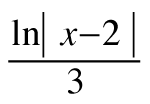and f(−3) =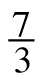. Homework Help ✎

1. Estimate f(−3.1).

2. Find f ″(x).

3. Use concavity to determine if your answer to part (a) is an under or over estimate of the actual value of f(x). Justify your answer.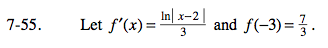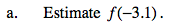Do NOT find an f(x) function. That would be tedious and unnecessary work.

This is another way of wording a very familiar problem:
'Given f '(x) and a point on f(x), find the tangent line to f(x) at x = −3 and use it to estimate the value of f(−3.1).'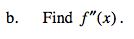Split the absolute value into a piecewise function and differentiate.
Or, you could recall a special note about the antiderivative of x−1: While you can generally leave it as ln(x), it actually equals ln|x|.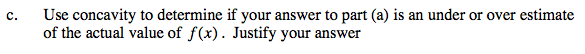If a function is concave down, all tangent lines will be above the curve.
If a function is concave up, all tangent lines will be below the curve. Sketch this to verify.